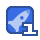# understanding machine learning15收藏

Machine learning is one of the fastest growing areas of computer science, with far-reaching applications. The aim of this textbook is to introduce machine learning, and the algorithmic paradigms it offers, in a principled way. The book provides an extensive theoretical account of the fundamental ideas underlying machine learning and the mathematical derivations that transform these principles into practical algorithms. Following a presentation of the basics of the field, the book covers a wide array of central topics that have not been addressed by previous textbooks. These include a discussion of the computational complexity of learning and the concepts of convexity and stability; important algorithmic paradigms including stochastic gradient descent, neural networks, and structured output learning; and emerging theoretical concepts such as the PAC-Bayes approach and compression-based bounds. Designed for an advanced undergraduate or beginning graduate course, the text makes the fundamentals and algorithms of machine learning accessible to students and non-expert readers in statistics, computer science, mathematics, and engineering.

...展开详情

• 2
资源
• 0
粉丝
•等级understanding machine learning 9积分/C币 立即下载
1/0